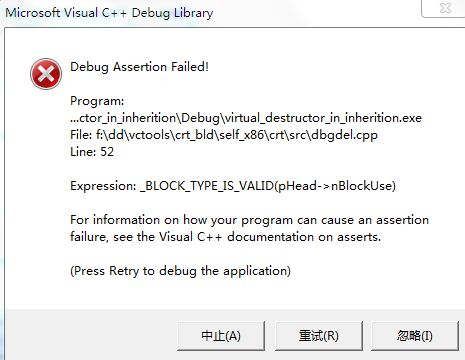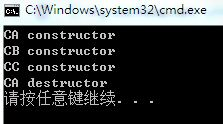## 继承中构造函数和析构函数的调用顺序 原

``````#include <iostream>

using namespace std;

class CA
{
public:
CA(){ cout << "CA constructor" << endl; }
~CA(){ cout << "CA destructor" << endl; }
};

class CB:public CA
{
public:
CB(){ cout << "CB constructor" << endl; }
~CB(){ cout << "CB destructor" << endl; }
};
class CC:public CB
{
public:
CC(){ cout << "CC constructor" << endl; }
~CC(){ cout << "CC destructor" << endl; }
};

int main(int argc, char* argv[])
{
CC c;
return 0;
}``````

CA是爷爷，CB是爸爸，CC是儿子。

``````CA constructor
CB constructor
CC constructor
CC destructor
CB destructor
CA destructor``````

(2) 再做第二个试验之前，先做一点小小修改

``````//~CA(){ cout << "CA destructor" << endl; }
virtual ~CA(){ cout << "CA destructor" << endl; }``````

``````int main(int argc, char* argv[])
{
CA* pt = new CC;
delete pt;

return 0;
}``````

yeah

``````CA constructor
CB constructor
CC constructor
CC destructor
CB destructor
CA destructor``````

``````CA constructor
CB constructor
CC constructor
CA destructor``````

(3)

``````int main(int argc, char* argv[])
{
CB * pt = new CC;
delete pt;

return 0;
}``````

``````CA constructor
CB constructor
CC constructor
CC destructor
CB destructor
CA destructor``````

``````class CA
{
public:
CA(){ cout << "CA constructor" << endl; }
~CA(){ cout << "CA destructor" << endl; }
};

class CB:public CA
{
public:
CB(){ cout << "CB constructor" << endl; }
~CB(){ cout << "CB destructor" << endl; }
};``````

``````CA constructor
CB constructor
CC constructor
CB destructor
CA destructor``````

``````class CA
{
public:
CA(){ cout << "CA constructor" << endl; }
~CA(){ cout << "CA destructor" << endl; }
};

class CB:public CA
{
public:
CB(){ cout << "CB constructor" << endl; }
virtual ~CB(){ cout << "CB destructor" << endl; }
};

class CC:public CB
{
public:
CC(){ cout << "CC constructor" << endl; }
~CC(){ cout << "CC destructor" << endl; }
};

int main(int argc, char* argv[])
{
CB * pt = new CC;
delete pt;

return 0;
}``````

3 中代码运行如下

``````CA constructor
CB constructor
CC constructor
CC destructor
CB destructor
CA destructor``````

(4)

``````int main(int argc, char* argv[])
{
CA * pt = new CC;
delete pt;

return 0;
}``````

``````class CA
{
public:
CA(){ cout << "CA constructor" << endl; }
~CA(){ cout << "CA destructor" << endl; }
};

class CB:public CA
{
public:
CB(){ cout << "CB constructor" << endl; }
virtual ~CB(){ cout << "CB destructor" << endl; }
};

class CC:public CB
{
public:
CC(){ cout << "CC constructor" << endl; }
~CC(){ cout << "CC destructor" << endl; }
};

int main(int argc, char* argv[])
{
CA * pt = new CC;
delete pt;

return 0;
}``````

（一） VC2010会出现提示内存错误CA constructor
CB constructor
CC constructor

CA desstructor

（二）GCC 只会调用CA的析构函数(5)
CA CB CC

``````int main(int argc, char* argv[])
{
void * pt = new CC;
delete pt;

return 0;
}``````

CA constructor
CB constructor
CC constructor

class A
{
public:
A()
{
cout<<"A()"<<endl;
}
};

class B:public A
{
public:
B()
{
cout<<"B()"<<endl;
}
};

class C
{
public:
C()
{
cout<<"C()"<<endl;
}
B  a;

};
int main(void)
{

C c;
return 0;
}

A()
B()
C()

C1 * p = new C2();
delete p;

delete p的时候，那么有以下几种情况：
1) C1

2
3
）如果C1void，那就什么析构都不调用了。### 评论(0)

C++ 类(继承中的构造和析构)

03/31
0
0
【C++温故】(2) 类的继承（一）

zizi7
03/31
0
0

sdoyuxuan
2017/04/07
0
0

2018/01/31
0
0
C++中继承及virtual小结

sinat_27652257
03/31
0
0

31分钟前
8
0

1、面向对象思想设计原则 在实际的开发中，我们要想更深入的了解面向对象思想，就必须熟悉前人总结过的面向对象的思想的设计原则 1.1、单一职责原则 高内聚，低耦合 每个类应该只有一个职责，...

39分钟前
25
0

40分钟前
5
0

//有一个容器，能存储一定的产品，有put和get方法，有两个生产者，8个消费者的线程阻塞 import java.util.LinkedList; import java.util.concurrent.TimeUnit; public class Test3<T> { Lin...

50分钟前
13
0

lihua20103181
52分钟前
100
0